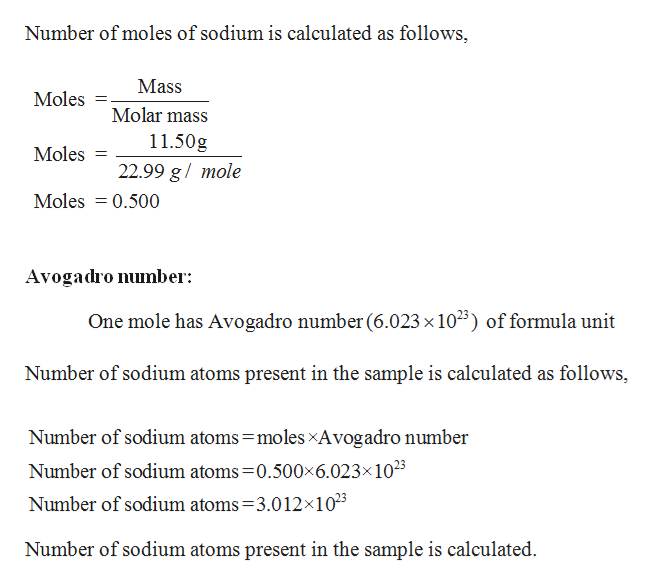# Suppose you have a sample of sodium weighing 11.50 g. How many atoms of sodium are present in the sample? What mass of potassium would you need to have the same number of potassium atoms as there are sodium atoms in the sample of sodium?

Question
65 views
Suppose you have a sample of sodium weighing 11.50 g. How many atoms of sodium are present in the sample? What mass of potassium would you need to have the same number of potassium atoms as there are sodium atoms in the sample of sodium?

check_circle

Step 1

Given,

Sodium weight 11.50 g

Molar mass o...help_outlineImage TranscriptioncloseNumber of moles of sodium is calculated as follows, Mass Moles Molar mass 11.50g Moles 22.99 g/ mole Moles 0.500 Avogadro number One mole has Avogadro number (6.023 x 103) of formula unit Number of sodium atoms present in the sample is calculated as follows, Number of sodium atoms moles xAvogadro number Number of sodium atoms 0.500x6.023x1023 Number of sodium atoms =3.012x1025 Number of sodium atoms present in the sample is calculated. fullscreen

### Want to see the full answer?

See Solution

#### Want to see this answer and more?

Solutions are written by subject experts who are available 24/7. Questions are typically answered within 1 hour.*

See Solution
*Response times may vary by subject and question.
Tagged in

### General Chemistry